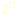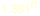Algorithms Syllabus Textbook: Thomas H. Cormen, Charles E. Leiserson, Ronald L. Rivest, Clifford Stein: Introduction to algorithms, MIT Press, Cambridge, Massachusetts and McGraw-Hill, New York, 2001. Instructor: János Pach Grading: 25% homework, 25% midterm test, 50% final exam. (1) Introduction: Easy and difficult problems, the "combinatorial explosion", intractable problems. Analyzing algorithms, asymptotic behavior of functions: polynomial vs. exponential. (2) Sorting problems: Bubble Sort, Insertion Sort and Merge Sort. Recursive, divide-and-conquer algorithms. Worst case complexity, recurrence relations. (3) Lower bounds for the sorting problem and for some other combinatorial searching problems. The decision tree model. (4) Quicksort. Worst case and average case analysis. Randomized algorithms. (5) Sorting in linear time (Counting Sort, Radix Sort, Bucket Sort). Finding the largest (smallest) and second largest elements. Finding the median (the Blum, Floyd, Pratt, Rivest, Tarjan algorithm). (6) Graphs, clique number, independence number, connectivity, the complement of a graph. Adjacency matrix. Matching and packing problems that can be reduced to the problem of finding the independence number. Recursive algorithms to solve this problem in timeand. Interval packing in linear time. (7) Breadth-first search, depth-first search. Shortest path algorithms: Dijkstra's algorithm, Ford's algorithm. Suboptimal algorithms (e.g., greedy algorithm for packing problems). (8) Minimum spanning trees, Kruskal's algorithm, Prim's algorithm. The number of spanning trees. Prüfer's code. (9) Maximum flow in a network. Kirchhoff's current law, cuts. The Ford-Fulkerson algorithm. Corollaries: Finding a maximum matching in a bipartite graph, determining the connectivity of a graph. (10) Epilogue: Multiplying faster. Midterm 1 ( ps, pdf ) Midterm 2 ( ps, pdf ) Midterm 3 + Solutions ( ps, pdf ) Midterm 4 + Solutions ( ps, pdf ) Final Assignment ( jpg ) Final exam ( jpg ) Final exam 2 ( jpg ) Final exam 3 ( jpg )